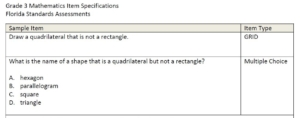What attributes should we use to describe quadrilaterals?

Define shapes using the following attributes: number of sides, number of angles, whether the shape has a right angle, whether the sides are the same length, and whether the sides are straight lines. (Note – it is not required that students identify acute and obtuse angles within the shapes. It is also not required to use the language parallel or perpendicular at this time).

Sample problem from FSA item specifications:What are the different names of quadrilaterals?

We define quadrilaterals using the following attributes:
• Quadrilateral – Polygon with 4 sides
• Parallelogram – 2 sets of sides of the same length
• Rectangle – 2 sets of sides of the same length and 4 right angles
• Rhombus – 4 equal sides
• Square – 4 equal sides and 4 right angles

Can a quadrilateral shape have more than one name?

The answer is, YES! Based on attributes listed, quadrilaterals can have more than one name. Sometimes we use the most inclusive name to identify the shape, but students need to understand that it can be named more than one way.

We need students to understand that shapes in different categories may share attributes. For example: rhombuses and rectangles share the attribute of 4 sides.
Because of that shared attributes, it can be used to define a larger category. For example: Because rhombuses and rectangles share the attribute of 4 sides that are in the quadrilateral category.

All the names for this shape are: square, rectangle, rhombus, parallelogram and quadrilateral.We want to encourage students to justify why it can be all the names using the attributes defined. An explanation to go with the example above could be: “I know a square can also be called a rectangle because to be a rectangle you must have 2 sets of sides of the same length and 4 right angles. A square shares these attributes, therefore a square can be called a rectangle. A rectangle is not a square because to be a rectangle you must have 4 equal sides and a rectangle does not.”

As students are discovering how shapes can be named, create an anchor chart that they can refer to when discussing quadrilaterals. Some example could include:Good tasks to encourage this discovery:
• Pose a shape like the one listed below and have students list all the names for the shape and justify why they fit.• Eliminate It! Justify why. Pose the four shapes and have students eliminate one then, then explain why using attributes and comparison.Sample problem from FSA item specifications website:How does comparing and contrasting attributes of quadrilaterals help deepen understanding?
• Concept Card – created by posing examples and non-examples of a specific quadrilateral. From the examples and non-examples, students determine a definition for the topic. This can be used for any geometry vocabulary word, including different types of quadrilaterals.• Venn Diagram & Power of Polygon shapes – Used to compare and contrast attributes of polygons or quadrilaterals. Consider this activity: Give the students a triple venn diagram and the attribute labels of polygon, quadrilateral, right angles. Have students sort their shapes into the sections and explain why they were placed where they are. (Note – there is a paper copy of these located on the GCG).